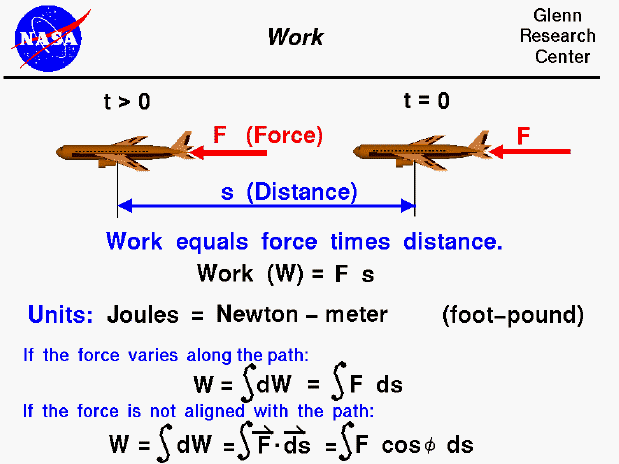Thermodynamics is a branch of physics which deals with the energy and work of a system. As mentioned on the gas properties slide, thermodynamics deals only with the large scale response of a system which we can observe and measure in experiments. As aerodynamicists, we are most interested in the thermodynamics of propulsion systems. The first law of thermodynamics deals with the relationship between heat and energy and work. We all have a basic understanding of work in general, but scientists have a more precise definition of work.

For scientists, work is the product of a force acting through a distance. As an example shown on the slide, an aircraft which is acted on by a force (F) from time (t) equals zero to some later time moves some distance (s). The work done on the aircraft during this time is F times s. The units of work is the Joule, which is equal to a Newton - meter. (In the English system the unit of work is the foot - pound). In our simple example, the force is a constant value aligned with the displacement of the aircraft. In most real applications, the force varies along the flight path in both magnitude and direction. The work is then equal to the integrated value of the component of the force in the direction of the displacement with the angle between the force and displacement equal to phi. It is important to note that work is only done on (or by) the component of the force along the path. Using a cruising aircraft as another example, the lift is defined to be the force perpendicular to the flight path. Lift does no work on a cruising aircraft because the displacement is perpendicular to the force. Similarly, if an aircraft was stopped at the end of the runway with the brakes on and the engines at full throttle, the engines do no work on the aircraft because the displacement distance is zero.

In the previous examples, we have been concerned with the work done on (or by) a force applied to a solid object. For a gas, work is done when the volume of a gas is changed at some pressure since the pressure represents a force per unit area and the volume can be related to the surface area and a distance. Notice that to produce work, the volume must change.

Guided Tours